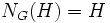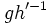# Free factor implies self-normalizing or trivial

Jump to: navigation, search
This article gives the statement and possibly, proof, of an implication relation between two subgroup properties. That is, it states that every subgroup satisfying the first subgroup property (i.e., free factor) must also satisfy the second subgroup property (i.e., self-normalizing subgroup)
View all subgroup property implications | View all subgroup property non-implications
Get more facts about free factor|Get more facts about self-normalizing subgroup

## Statement

Suppose$G = H * K$, i.e.,$G$ is a free product of subgroups$H$ and$K$, so$H$ is a free factor of$G$. Suppose further that$H$ is a nontrivial group. Then,$H$ is a self-normalizing subgroup of$G$:$N_G(H) = H$.

## Proof

Given: A free product$G = H * K$.

To prove:$H$ is self-normalizing in$G$.

Proof: Suppose$H$ is not self-normalizing in$G$. Pick any$g \in N_G(H) \setminus H$. Then we can write$g$ uniquely as an alternating product of elements from$H$ and$K$, with at least one letter from$K$. If the first letter for the unique expression for$g$ is$h \in H$, we can replace$g$ by$h^{-1}g$ to get a new element in$N_G(H)$ whose first letter is in$K$. Similarly, if the last letter is$h' \in H$, we can replace$g$ by$gh'^{-1}$ to get a new element in$N_G(H)$ whose last letter is in$K$. Thus, we can, without loss of generality, assume that:$g = k_1h_1 \dots k_{n-1}h_{n-1}k_n$

where each$h_i \in H, k_i \in K$, and all elements are non-identity elements. Now, consider any non-identity element$h \in H$ (we can do this because$H$ is a nontrivial group). Clearly, the word$ghg^{-1}$ is also a reduced word, and since this word has length more than one, we see that$ghg^{-1} \notin H$. This contradicts the assumption that$g \in N_G(H)$, completing the proof.